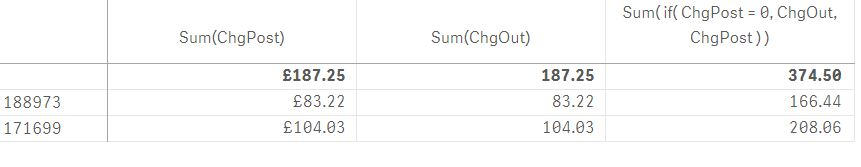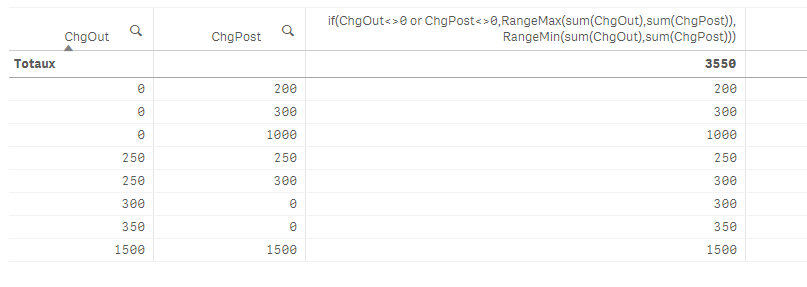# New to Qlik Sense

Discussion board where members can get started with Qlik Sense.

Announcements
Attend QlikWorld 2020 and hear keynote speaker, Malcolm Gladwell. Register by February 29th to save \$200. Learn More
Highlighted
New Contributor III

## Calculated dimension based on a value of one of the cells

Hello,

I need to create calculated dimension in the table as below:

 ChgPost ChgOut Calculated 1000 0 1000 1500 1500 1500 200 0 200 300 0 300 0 300 300 0 350 350 250 250 250 300 250 250

so Calculated = ChgPost  but if ChgPost = 0 then Calcuated = ChgOut.

I used the below formula:

If(ChgPost=0,ChgOut,ChgPost)

but it looks like the calculated = ChgPost+ChgOut

 ChgPost ChgOut Calculated 1000 0 1000 1500 1500 3000 200 0 200 300 0 300 0 300 300 0 350 350 250 250 500 300 250 550

Thanks,

Leszek

1 Solution

Accepted Solutions
HighlightedPartner

## Re: Calculated dimension based on a value of one of the cells

you can use the below expression in your measure:

Sum( if( ChgPost = 0, ChgOut, ChgPost ) )

OR

create a new field within your script as below:

...

if( ChgPost = 0, ChgOut, ChgPost ) as Calculated

and use Sum(Calculated) as your measure.

8 Replies
Highlighted
New Contributor III

## Re: Calculated dimension based on a value of one of the cells

NOt sure if I worded it correctly, I am trying to calculate a filed in the table in  Data Manager view. Please see below.Thank you for your help as always.

Thanks,

Leszek

HighlightedPartner

## Re: Calculated dimension based on a value of one of the cells

you can use the below expression in your measure:

Sum( if( ChgPost = 0, ChgOut, ChgPost ) )

OR

create a new field within your script as below:

...

if( ChgPost = 0, ChgOut, ChgPost ) as Calculated

and use Sum(Calculated) as your measure.

Highlighted
New Contributor III

## Re: Calculated dimension based on a value of one of the cells

HI Andrea,

thank you, I used the expression Sum( if( ChgPost = 0, ChgOut, ChgPost ) ) but the result looks like Calculated = ChgOut + ChgPost

Which is not what I wanted.

HighlightedPartner

## Re: Calculated dimension based on a value of one of the cells

for the row with 1500 in both fields value, you'll get 1500 as result not 3000 applying the above expression.

Highlighted
New Contributor III

## Re: Calculated dimension based on a value of one of the cells

that what I expected to see. i.e. calculated value to be 1500 but I get 3000?

Highlighted
New Contributor III

## Re: Calculated dimension based on a value of one of the cellsHighlighted
New Contributor III

## Re: Calculated dimension based on a value of one of the cells

I realised where the mistake is, I changed the expression to

if( Sum(ChgPost) = 0, Sum(ChgOut), Sum(ChgPost))

and it worked as I am looking at Sum of ChgPost not ChrgPost itself. Thank you for your help!Partner

## Re: Calculated dimension based on a value of one of the cells

if(ChgOut<>0 or ChgPost<>0,RangeMax(sum(ChgOut),sum(ChgPost)), RangeMin(sum(ChgOut),sum(ChgPost)))

result: